# DAV Class 7 Maths Chapter 8 Worksheet 1 Solutions

The DAV Maths Book Class 7 Solutions Pdf and DAV Class 7 Maths Chapter 8 Worksheet 1 Solutions of Triangle and Its Properties offer comprehensive answers to textbook questions.

## DAV Class 7 Maths Ch 8 WS 1 Solutions

Question 1.
Look at the figure carefully and complete the statements.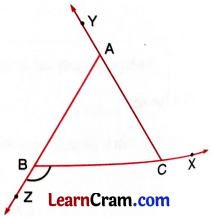(a) ∠BAY = ∠ABC +
∠ACB

(b) ∠CBZ = ∠____ + ∠______
∠BAC

(c) ___________ = ∠CAB + ∠ABC
∠ACBQuestion 2.
In the given figure, find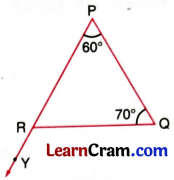(a) ∠YRQ
∠YRQ = ∠P + ∠Q
= 60° + 70°
= 130°

(b) ∠PRQ
∠PRQ = 180° – (60° + 70°)
= 180° -130°
= 50°

Question 3.
One of the exterior angles of a A ABC measures 150°. If one of the interior opposite angle is 75°, find the other interior opposite angles. What type of triangle is this?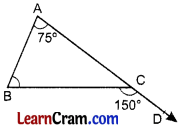Let the other exterior opposite angle be x°
Exterior angle BCD = 150°
∠A = 75°
∠BCD = ∠A + ∠B
150° = 75° + ∠B
∠B = 150° – 75° = 75
Hence ∠B = 75°
As ∠A = ∠B = 75°
∆ABC is an isosceles triangle.Question 4.
One of the exterior angles of a triangle is 100°. The interior opposite angles are equal to each other. Find the measure of these equal interior opposite angles.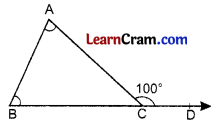Let A and B be two equal interior opposite angles for an exterior angle ACD
∠A = ∠B
∠ACD = ∠A + ∠B
100° = ∠A + ∠A [∠A = ∠B]
⇒ 100° = 2∠A
Hence ∠A = ∠B = 50°.

Question 5.
In a triangle one of the exterior angles is 105 75°. Find the measure of all the angles of the triangle.∠ACD = ∠A + ∠B [Exterior angle of a
=> 105° = ∠A + 75° triangle]
=> 105° – 75° = ∠A
∠A = 30°
Hence ∠A = 30°

Question 6.
In the given triangle, find the measure of ∠PRQ.Here, ∠RQS = 140°
∠RPQ = 90°
∠RQS = ∠P + ∠R [Exterior angle]
140° = 90° + ∠R
∠R = 140° – 90° = 50°
Hence ∠R = 50°.### DAV Class 8 Maths Chapter 8 Worksheet 1 Notes

Scalene Triangle:
The triangle which have its all sides of different length is called scalene triangle.
In the given Fig. 8.1, AB, BC and AC are different.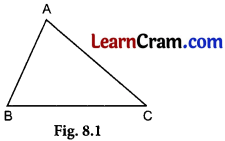Isosceles Triangle:
The triangle in which any two sides are equal. In the given Fig. 8.2,
AB = AC, BC is the base and ¿B – ¿C.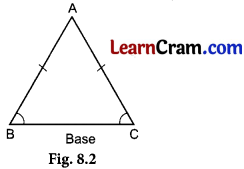Equilateral Triangle:
The triangle whose all sides and all angles are equal. AB = AC = BC and
∠A = ∠B = C (Fig. 8.3).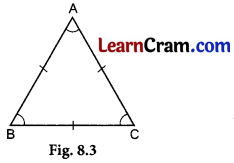Exterior Angle:• Exterior angle of a triangle is equal to the sum of its interior opposite angles.
• In Fig. 8.4, ∠ACD is exterior angle and ∠ACD = ∠A + ∠B.
• Exterior angle is always greater than its interior opposite angles.
• Sum of the angles of a triangle is 180°. (Angle sum property of a triangle).
• The sum of the lengths of any two sides of a triangle is greater than the third side. Triangle inequality.Pythagoras Theorem:
In a right triangle, the square of the hypotenuse is equal to the sum of the squares of its other two sides.
In Fig. 8.5, ∠B = 900, AC2 = AB2 + BC2Converse of Pythagoras Theorem:
If the square of the longer side is equal to the sum of the squares of other two sides, then the angle opposite to the longer side is 90°.
In a right triangle, the hypotenuse is the longest side.

Median of a Triangle:

• A median of a triangle is a line segment joining a vertex to the mid-point of the side opposite to the vertex.
• In the given figure, D is the mid-point of the side BC of the ABC and A is the vertex opposite to it. So, AD is median from the vertex A.
• Since a triangle has three vertices, it will have three medians, one from each vertex.Centroid of a Triangle:
The point of intersection of the medians of a triangle is called the centroid of the triangle. Centroid may be inside or outside of the triangle, in Fig. (8.6) AE, BF and CD are the medians and O is the centroid.Altitude of a Triangle:

• An altitude of a triangle is a line segment drawn perpendicular from the vertex to the opposite side.
• In the given figure, ∆ABC is an acute angled triangle and AD is the altitude.
• Every triangle has three altitudes from each vertex.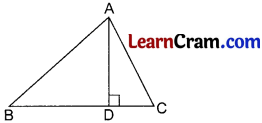Orthocentre of a Triangle:
The point of intersection of the altitudes of a triangle is called orthocentre. In Fig. (8.7) AD, BE and CF are the altitudes and O is the Orthocentre.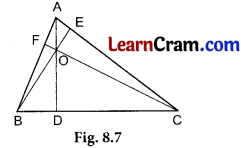Circumcentre of a Triangle:
The point of intersection of perpendicular bisectors of the sides of a triangle is called circumcentre. In Fig. (8.8) OD, EO and FO are the perpendicular bisectors of BC, AC and AB respectively, O is the circumcentrc.
The circurncircle passes through vertices of the triangle.Incentre of a Triangle:

• The point of intersection of the angle bisectors of a triangle is called incentre. In Fig. (8.9), AO, BO and CO are the angle bisectors and O is the incentre.
• Incentre always lies inside the triangle.
• The incircie touches each side of a triangle internally.
• Incentre is equidistant from the sides and circumcentre is equidistant from vertices of the triangle.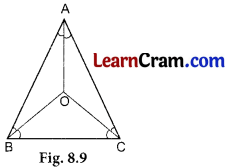Example 1:
In the given figure, ∠BCD = 130°, ∠B = 60°, find the measure of ∠A.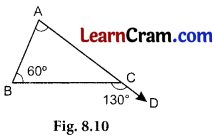∠BCD = ∠A + ∠B
(Exterior angle of a triangle is equal to the sum of its interior opposite angles]
⇒ 130° = ∠A + 60°
⇒ ∠A = 130° – 60°
∴ ∠A = 70°

Example 2:
In the given figure, ABC is an isosceless triangle in which AB = AC, ∠A = 80°, find ∠B and ∠C.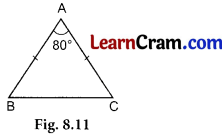In ABC, AB = AC.
∴ ∠B = ∠C [Base angles of an isosceles triangle]
∠A + ∠B + ∠C = 180° [Sum of the angles of a triangle is 1800]
⇒ 80° + ∠B + ∠B = 180°
⇒ 80° + 2∠B = 180°
⇒ 2∠B = 180° – 80°
⇒ 2∠B = 100°
⇒ ∠B = 50°
Hence ∠B = ∠C = 50°Example 3:
In the given figure, AB = AC, ∠A = 70°, find a and b.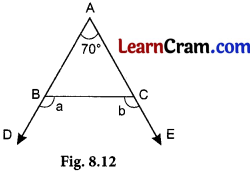AB = AC
∴ ∠B = ∠C [Angles opposite to equal sides are equal]
∠A + ∠B + ∠C = 180° [Angle sum property]
⇒ 70° + ∠B + ∠B = 180°
⇒ 2∠B = 180° – 70° = 110°
∴ ∠B = $$\frac{110}{2}$$ = 55°
⇒ ∠B = ∠C = 55°
⇒ a + ∠B = 180° [Linear Pair]
⇒ a + 55° = 180°
⇒ a = 180° – 55°
⇒ a = 125°
⇒ b + ∠C = 180°
⇒ b + 55° = 180° [Linear Pair]
⇒ b = 180° – 55° = 125° [Linear Pair]
Hence a = 125° and b = 125°.

Example 4:
In the given figure find the values of x and y.y + 120° = 180° (Linear Pair)
⇒ y = 180° – 120°
⇒ y = 60°
∠A + ∠B + ∠C = 180° [Angle sum propertyl
⇒ 50° + x + 60° = 180°
⇒ x = 180° – 50° – 60° = 70°
Hence x = 70° and y = 60°Example 5:
State which of the following are Pythagorean triplets.
(i) 8, 10, 6
(ii) 7, 8, 9
(iii) 20, 21, 29,
(iv) 5, 13, 12
(i) The triplet is 8, 10, 6 100 = 64 +36
(8)2 = 64,
(10)2 = 100,
(6)2 = 36
⇒ 100 = 64 + 36
⇒ 100 = 100
Hence 8, 10, 6 is a Pythagorean triplet.

(ii) The given triplet is 7, 8, 9
(7)2 = 49,
(8)2 = 64,
(9)2 = 81
⇒ 81 ≠ 49 + 64
⇒ 81 ≠ 113
Hence 7, 8, 9 is not a Pythagorean triplet.

(iii) The given triplet is 20, 21, 29
(20)2 = 400,
(21)2 = 441,
(29)2 = 841
⇒ 841 = 400 + 441
⇒ 841 = 841
Hence 20, 21, 29 is a Pythagorean triplet.

(iv) 5, 13, 12 is the given triplet
(5)2= 25,
(13)2 = 169,
(12)2 = 144
⇒ 169 = 25 + 144
⇒ 169 = 169
Hence 5, 13, 12 is a Pythagorean triplet.Example 6:
ABC is a right triangle in which ∠B = 90°. If AB = 7 cm and BC = 24 cm, find AC.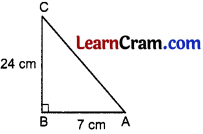In Δ ABC, ∠B = 90°
∴ AB2 + BC2 = BC2 (Pythagoras Theorem)
⇒ AC2 = (7)2 + (24)2
⇒ AC2 = 49 + 576
⇒ AC2 = 625
AC = $$\sqrt{625}$$
∴ AC = 25
Hence AC = 25 cm.

Example 7:
Find the measure of all the exterior angles of a triangle ABC.Let the three exterior angles of Δ ABC be x°, y° and z° respectively.
∴ ∠x = ∠B + ∠C (Exterior angle) ……………..(i)
Similarly ∠y = ∠A + ∠C ………….(ii)
and ∠z = ∠A + ∠B ………………(iii)
Adding (i), (ii) and (iii) we get,
∠x + ∠y + ∠z = 2∠A + 2∠B + 2∠C
= 2(∠A + ∠B + ∠C)
= 2 × 180° (Angle Sum Property)
Hence the sum of all the exterior angles is 360°.Example 8.
In ABC, ∠A = (2x + 15)°, ∠B = (3x – 20)° and ∠C = (x + 65)°, find x.
⇒ x = $$\frac{120}{6}$$ = 20°